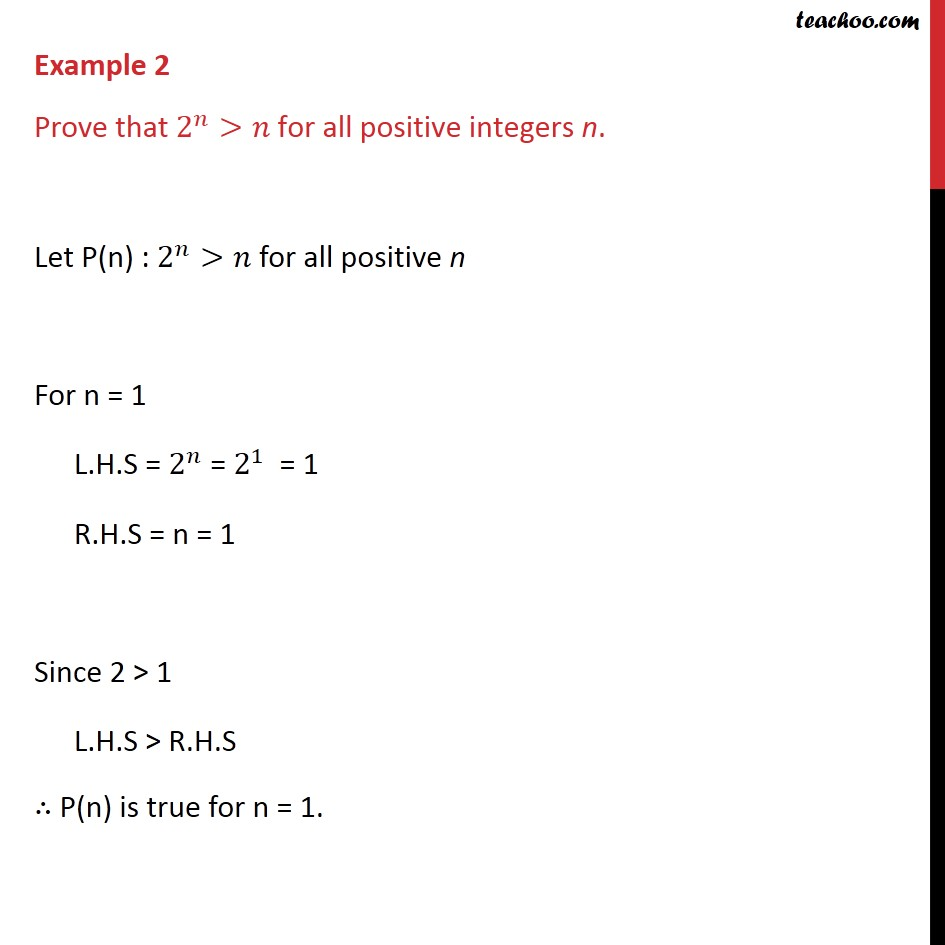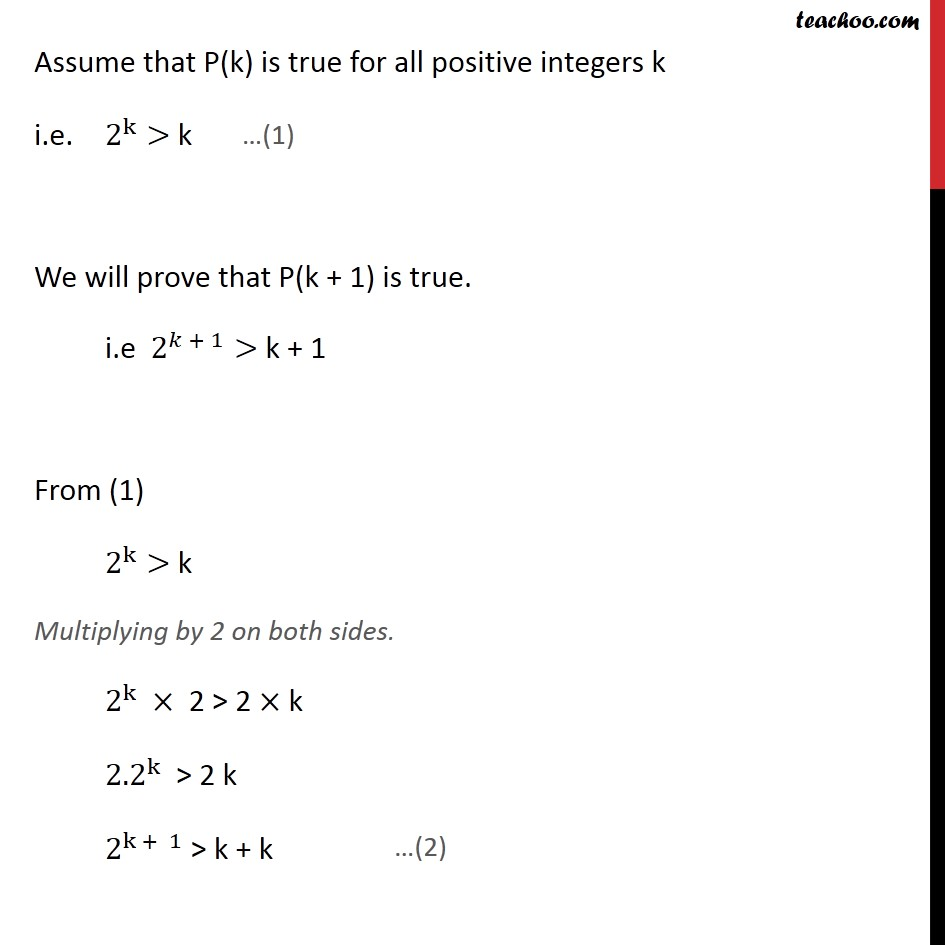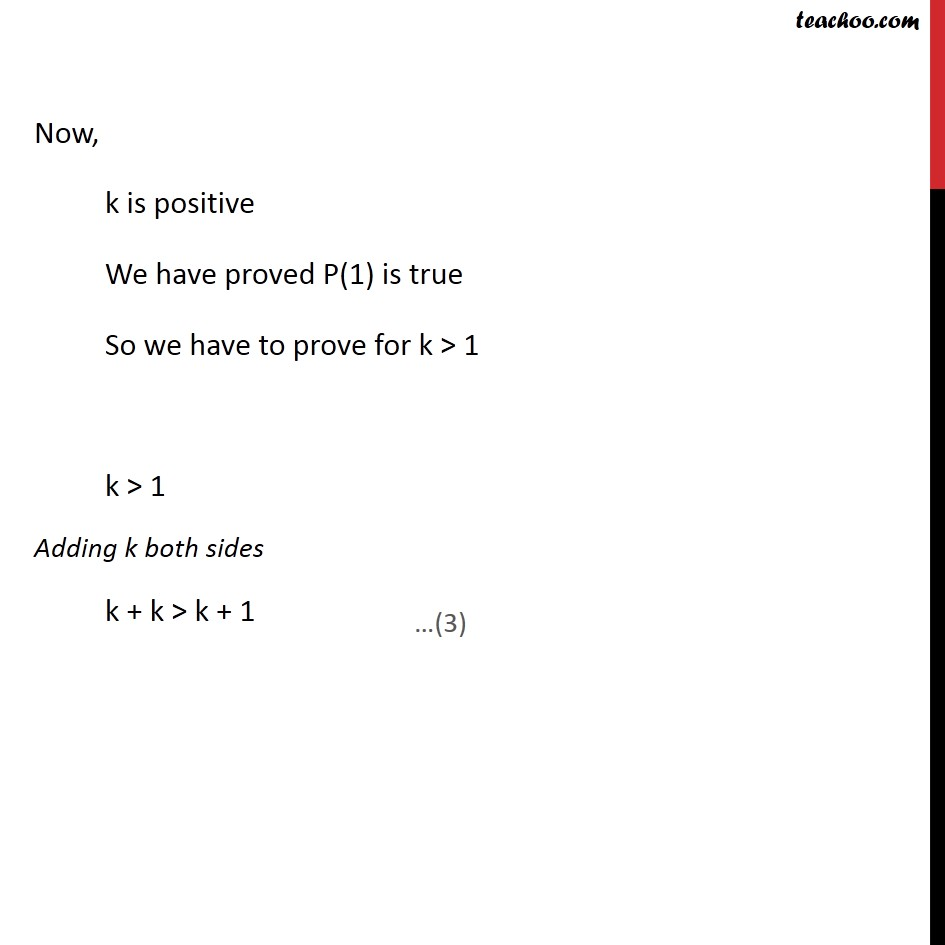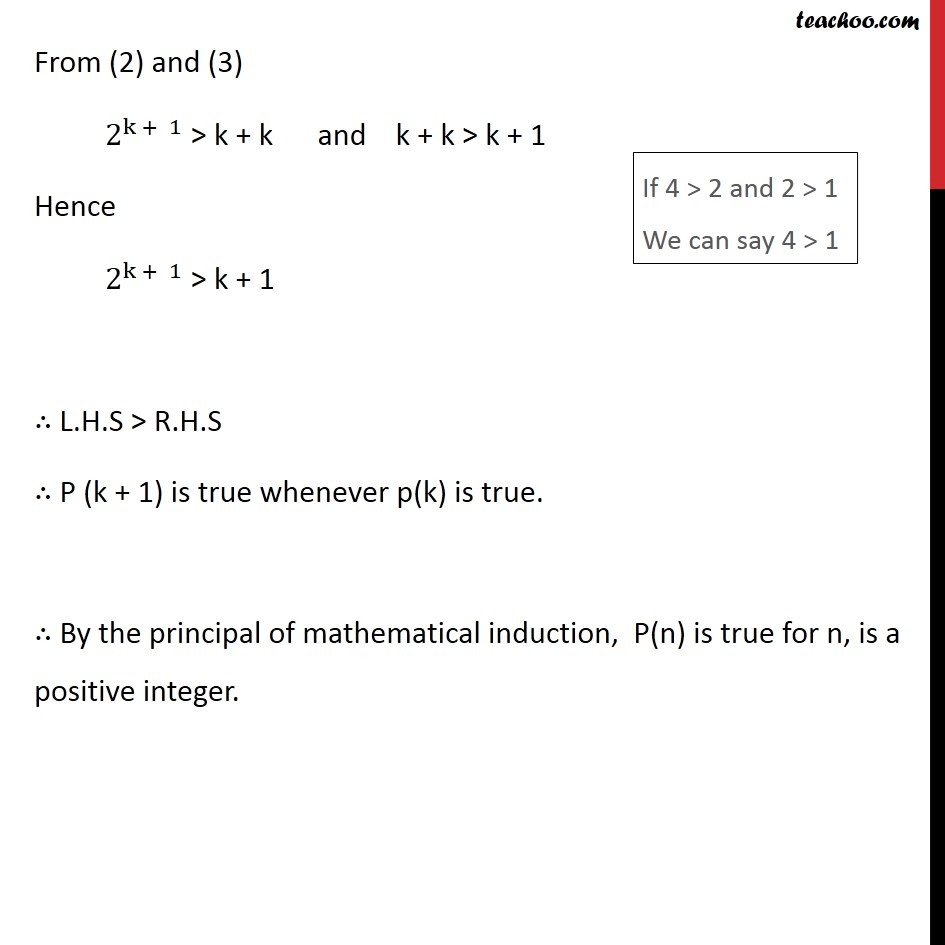Examples

Chapter 4 Class 11 Mathematical Induction (Deleted)
Serial order wise983 students joined Teachoo Black. What are you waiting for?

### Transcript

Example 2 Prove that 2﷮𝑛﷯>𝑛 for all positive integers n. Let P(n) : 2﷮𝑛﷯>𝑛 for all positive n For n = 1 L.H.S = 2﷮𝑛﷯ = 2﷮1﷯ = 1 R.H.S = n = 1 Since 2 > 1 L.H.S > R.H.S ∴ P(n) is true for n = 1. Assume that P(k) is true for all positive integers k i.e. 2﷮k﷯> k We will prove that P(k + 1) is true. i.e 2﷮𝑘 + 1﷯> k + 1 From (1) 2﷮k﷯> k Multiplying by 2 on both sides. 2﷮k﷯ × 2 > 2 × k 2.2﷮k﷯ > 2 k 2﷮k + 1﷯ > k + k Now, k is positive We have proved P(1) is true So we have to prove for k > 1 k > 1 Adding k both sides k + k > k + 1 From (2) and (3) 2﷮k + 1﷯ > k + k and k + k > k + 1 Hence 2﷮k + 1﷯ > k + 1 ∴ L.H.S > R.H.S ∴ P (k + 1) is true whenever p(k) is true. ∴ By the principal of mathematical induction, P(n) is true for n, is a positive integer.